Uses of Left Right Mid Text Functions in Excel

Why To Use Left Function in Microsoft Excel?

The Left function is used to get first N characters from the given text string or the text contained in a cell.

Syntax of LEFT Function in Excel

LEFT(Text, Number_of_characters)

For example, the function LEFT("Normal",3) will return Nor.

Syntax of LEFT function with Cell Reference

LEFT(CellReference, Number_of_characters)

For example, let the cell A2 contains the text Humanity, the function LEFT(A2,5) will return Human.
Similarly if Cll A12 contains "England" then Left(A12,5) will give "Engla" that is first five characters of contents of cell A12 from left side.

How To Implement LEFT Function in Excel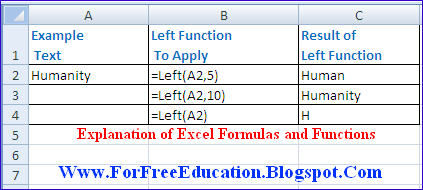Uses of Excel Functions Left Right in Excel Worksheet
First of all place the cursor in an empty cell next to cell A2, to enter the function.
• Type =
• Type the function name LEFT
• Type the left parenthesis (
• Type A2 from keyboard.
• Type , (that is Comma)
• Type 5 so that the first 5 characters will be returned from cell A2's text.
• Press Enter key (You need not to enter right parenthesis, it will be automatically included)
• The result Human will be displayed in the cell.
Note: Number_of_characters will be normally greater than zero. If Number_of_characters will greater than the total length of the text then All the text will be returned. For example, in above example the function LEFT(A2, 10) will return the whole text in cell A2 that is Humanity.
Similarly, if we ignore the value of Number_of_characters then it is assumed to be 1 by default. So that the function LEFT(A2) will return only one character that is H.

Why To Use Right Function in Microsoft Excel?

The Right function is used to get the last N characters in a text string. Where N is the number of characters to be extracted from the given string.

Syntax of RIGHT Function in Excel

RIGHT(Text, Number_of_characters)

For example, the function Right("Night",2) will return ht.

Syntax of RIGHT function with Cell Reference

RIGHT(CellReference, Num_of_char)

For example, let the cell A2 contains the text Saturday, the function RIGHT(A2,3) will return day.

How To Implement RIGHT Function in Excel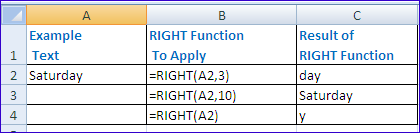First of all place the cursor in an empty cell next to cell A2, to enter the function.
• Type =
• Type the function name RIGHT
• Type the left parenthesis (
• Type A2 from keyboard.
• Type , (that is Comma)
• Type 3 so that the last 3 characters will be returned from cell A2's text.
• Press Enter key (You need not to enter right parenthesis, it will be automatically included)
• The result day will be displayed in the cell.
Note:
• Num_of_char will be greater than zero normally.
• If Num_of_char is greater than the length of given text, then RIGHT function will return all of text.
• If the value of Num_of_char is not used then its default value 1 is used and only one last character is returned by the RIGHT function.

Why To Use MID Function in Microsoft Excel?

The MID function is used to get the last N characters in a text string. Where N is the number of characters to be extracted from the given string.

Syntax of RIGHT Function in Excel

MID(text, start_num, num_chars)

For example, the function MID("Night",1,3) will return Nig.

Syntax of RIGHT function with Cell Reference

RIGHT(CellReference, start_num, num_chars)

Where start_num is the number of starting character and num_chars is total number of characters to be extracted from the text.
For example, let the cell A2 contains the text Reservation,
• the function MID(A2,6,3) will return vat.
• the function MID(A2,6,10) will return vation. Since 10 is greater than the length of 6 th character to end of text, so this MID function will return all text starting from 6th character v to last character n.
• the function MID(A2,1,1) will return only one character starting from position 1 that is R.

How To Implement RIGHT Function in Excel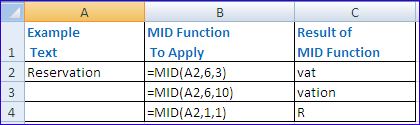First of all place the cursor in an empty cell next to cell A2, to enter the function.
• Type =
• Type the function name MID
• Type the left parenthesis (
• Type A2 from keyboard.
• Type , (that is Comma)
• Type 6
• Type ,
• Type 3 so that the 3 characters from position 6 will be returned cell A2's text.
• Press Enter key (You need not to enter right parenthesis, it will be automatically included)
• The result vat will be displayed in the cell.

Learn Practical Use of MAX Function and MIN Function in Excel Worksheets

What is MAX Function?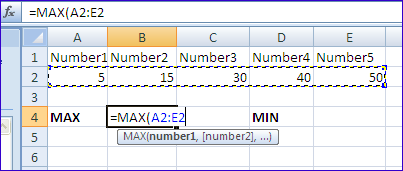Learn Practical Use of MAX Function and MIN Function in Excel Worksheets
The MAX function is used to get the maximum number from different given numbers in Excel Worksheet.

Syntax of MAX Function in Excel

MAX(number1,number2,number3,...numberN)
For example the function MAX(5,100,35) will return 100, since 100 is largest number of all 5, 100 and 35.

Syntax of MAX Function using Cell References

MAX(CellRef1:CellRef2)
Where CellRef1 is the cell reference of the first cell and CellRef2 is the cell reference of the last cell in the given cell range in Excel Worksheet.

What is MIN Function?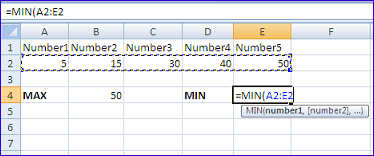The MIN function is used to get the minimum number from different given numbers in Excel Worksheet.

Syntax of MIN Function in Excel

MIN(number1,number2,number3,...numberN)
For example the function MIN(5,100,35) will return 5, since 5 is the smallest number of all 5, 100 and 35.

Syntax of MIN Function using Cell References

MIN(CellRef1:CellRef2)
Where CellRef1 is the cell reference of the first cell and CellRef2 is the cell reference of the last cell in the given cell range in Excel Worksheet.

How To Implement MAX Function in Excel

First of all place the cursor in an empty cell to enter the function.
• Type =
• Type the function name MAX
• Type the left parenthesis (
• Select the cell range A2 to E2 with the help of mouse so that the arguments A2:E2 will be automatically entered in the MAX function.
• Note: that you may type A2:E2 by keyboard also.
• Press Enter key (You need not to enter right parenthesis, it will be automatically included)
• The result 50 will be displayed in the cell.

How To Implement MIN Function in Excel

First of all place the cursor in an empty cell to enter the function.
• Type =
• Type the function name MIN
• Type the left parenthesis (
• Select the cell range A2 to E2 with the help of mouse so that the arguments A2:E2 will be automatically entered in the MIN function.
• Note: that you may type A2:E2 by keyboard also.
• Press Enter key (You need not to enter right parenthesis, it will be automatically included)
• The result 5 will be displayed in the cell.

Example Worksheet Picture For MAX and MIN FunctionsPractical Use of Max Function and MIN Min Function in Excel Worksheet

Use of Average Function in Excel Worksheet With Examples

What is the Use of Average Function in Excel?

The Average Function of Excel is used to get the average of the numbers in Excel Worksheet.

Syntax of Average Function in Excel

Average(number1, number2, number3, ... number N)For Example Average(10,15,20) will return 15. Because, Average is calculated by adding all the numbers and then dividing the total by count of the numbers.
In above example: Average = (10+15+20) / 3 Which evaluates to 45 / 3 that is 15.

Syntax of Average Function in Excel Using Cell references

Average(cellRef1:CellRef2)
where cellRef1 is the cell reference of the first cell and cellRef2 is the cell reference of the last cell in Average function to calculate the required average, in Excel worksheet.Consider the above figure for example: the cells A2, B2, C2, D2 and E2 contains the numbers 5, 15, 30, 40, 50 respectively.

How To Implement Average Function in Excel

First of all place the cursor in an empty cell to enter the function.
• Type =
• Type the function name AVERAGE
• Type the left parenthesis (
• Select the cell range A2 to E2 with the help of mouse so that the arguments A2:E2 will be automatically entered in the AVERAGE function.
• Note: that you may type A2:E2 by keyboard also.
• Press Enter key (You need not to enter right parenthesis, it will be automatically included)
• The result 28 will be displayed in the cell.

Explain Different Uses of Excel in Business and Commerce

Microsoft Excel is very useful in Business and Commerce. Following is a list of the uses of Microsoft Excel in Business and Commerce:1. Creating Computerized Worksheets

Microsoft Excel is a computer software with electronic worksheets. You can easily input, edit, format your business data in computerized worksheets provided by MS Excel. You can manipulate business data easily and efficiently.

Microsoft Excel can be used in business and commerce for recording the daily transaction records of your business. It provides the facility to store and maintain large amounts of records easily and quickly.

a) Accounting System

Microsoft Excel can be used to manage the accounting system of your business organization.It records daily transactions of the organization.

b) Prepare Accounting Documents

Microsoft Excel can be used to prepare balance sheet, trial balance, ledger and other accounting sheets.

4. Maintaining Stock System

Microsoft Excel can be used to record the stock of products and materials for a business organization. In addition Excel provides many functions to perform quick calculations for stock records.

5. Graphical Representation of Data

Microsoft Excel provide rich facilities to represent data graphically. Excel uses its Charts feature to represent data in interesting and colorful way so that the users can understand the facts and figures easily and quickly.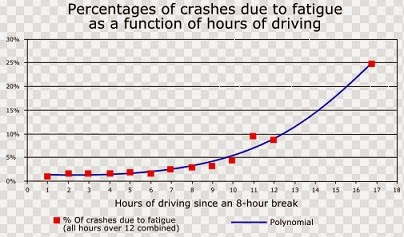6. Predefined Functions

Microsoft provides a feature rich set of built-in functions including mathematical functions, statistical functions, logical functions, text functions and date and time functions etc. These functions make the large and complex calculations very easy and quick.How to Use Predefined Functions of Microsoft Excel

7. Quick Calculations

Microsoft Excel provides different mathematical and other operators and predefined functions to apply different formulas on data.Quicker Calculations in Microsoft Excel

8. Automatic Recalculation

Microsoft provides the popular Automatic Recalculation feature. If you change the data then the results of the formulas will be automatically recalculated.

Practical Use of SUM Function in Excel Worksheet

What is SUM Function?

The SUM Function is used to add all numbers in a given range of cells and returns the total as a result. The syntax of the SUM Function is as follows:

SUM(FirstCell : LastCell)

where FirstCell is the cell reference of the first cell in cell range and LastCell is the cell reference of the last cell in the given cell range to be added.
For Example: If cells A1,A2,A3,A4 contains 10,20,30,40 respectively then the function =SUM(A1:A4) will give 100.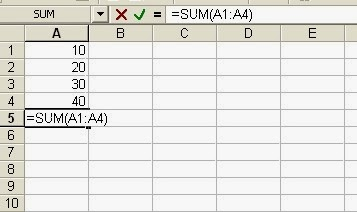Simple Use of SUM function in MS Example with examples

Syntax 2 of SUM Function in Excel Worksheet:

SUM(number1, number2, number3, ..., number N)
For Example:
= SUM(10,20,30)
will give the result 60.   and  = SUM(2,5) will give 7.

Syntax 3 of SUM Function in Excel Worksheets:

SUM(cell1, cell2, cell3, . . ., cell N)
Where cell1, cell2, cell3,...cell N are the cell references of the cells containing numbers to be added.
For Example: =SUM(A1, A3,A5) will give 10 if cell A1, A3, A5 have 2,3,5 respectively.There are some more possibilities to use the SUM function as following: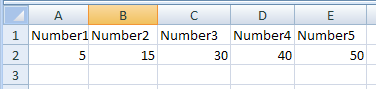If cells A2:E2 contain 5, 15, 30, 40, and 50 as show in above Excel Worksheet image:
SUM(A2:C2) equals 50        because 5+15+30
and SUM(B2:E2, 15) equals 150    because 15+30+40+50   and +15

If A1:A4 contains 10,20,30,40 respectively and cell A5 contains a non numeric value like "Excel" then the function = SUM(A1:A5) will return 100 because it ignores non-numeric value in Cell A5 that is Excel.

Explain Different Features of Microsoft Excel

Microsoft Excel is a spreadsheet software developed by Microsoft Corporation of USA. Microsoft Excel is used to input and format data and to apply suitable mathematical and other formulas on the data. Excel can perform large number of calculations on data easily in a table like format. The main file of Microsoft Excel is called an Excel Workbook. By default, each workbook has three Excel Worksheets. A Worksheet is divided into Rows, Columns and cells. Microsoft Excel provides many easy to use features as follows:

Important Features Provided by MS Excel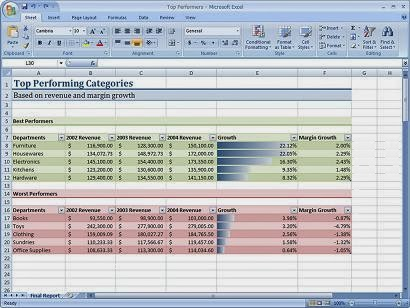different features of Microsoft Excel application software

1.    Auto Fill

MS Excel provides easy series generating feature called Auto Fill. With the help of Auto Fill you can fill the adjacent cell range with a series like days of a weak or a number series like 1,2,3,4,...10 or 5,10,15,...50 or 1990, 1991, 1992... or dates etc.Header and footer feature is used to display same text on top and bottom of each page. For example the information like page numbers, date written, author name, chapter name etc. can be written in header or footer.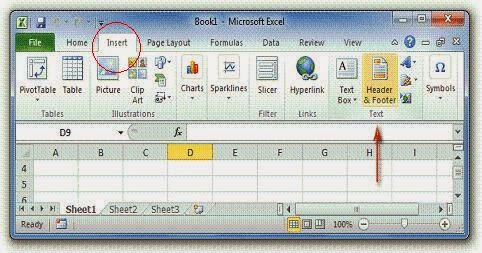3.    Easy Formatting

The text and numeric data can be easily formatted in Microsoft Excel. For example, the font style, font size, font color and text alignment in cells can be easily changed.4.    Spelling and Grammar Check

Spelling and Grammar feature is used to help you to correct spelling and grammar errors. Spelling errors are underlined by red wavy line and the errors in Grammar are highlighted by green wavy line.Misspelled words can be correct by just clicking right button of mouse on the word, a menu with spelling suggestions will appear, select the suitable word, as shown in the figure above.

5.    Auto Correct

Auto Correct feature is used to correct many common misspelled words and punctuation marks.

6.    The Formula Bar

It is used to enter different formulas for calculations. For example, if we are to add three numbers then we will type the formula "=A1+A2+A3" in the cell of worksheet.7.    Built-in Functions

Excel has many built-in functions. You can use functions to apply lengthy calculations easily.For example, if we have to calculate total of first then cells from A1 to A10 then the formula is:
= A1+A2+A3+A4+A5+A6+A7+A8+A9+A10
This formula may be very very long if we are to take total of A1 to A100 hundred cells. Therefore we use built in functions like SUM function. We will type "=SUM(A1:A100)" in the cell and get the resultant total.8.     Sorting

Sorting feature is used to sort data in ascending or descending order. For Example we will sort Roll Number column in ascending order from 1,2,3 ... and so on. On the other hand, we may chose to sort the percentage of marks obtained by students in Descending order to prepare a Merit List.

9.    Charts

Excel provides chart feature to present data in the form of charts and graphs for easy and graphical representation. In Microsoft Excel 2007 different types of charts can be created for numerical data stored in worksheets. Charts make it easy to compare and understand large amounts of numerical data. For example, consider the worksheet of a cricket match. If we record scores of the 11 batsmen of Cricket Team A as follows:
 S.No. Batsman Score 1 Inzamam 100 2 Afridi 50 3 Misbah 30 4 Shehzad 90 5 Nasir 2 6 Fahad 8 7 Kamran 35 8 Umer 25 9 Umer Gul 0 10 Saeed 12 11 Junaid 4
Note that we have to read whole table of names and scores if we want to find the highest or lowest scores by the batsmen. But looking at the chart created in Microsoft Excel, we can see the highest score by the highest column which is 100 scores of Inzamam.10.    Filter

Filter feature is used to see the required data according to some given criteria. In this way, we can see the needed data only, out of large amounts of data in worksheet. For Example we can apply top ten records filter to see only top ten students with highest marks in descending order.11.    Auto Complete

Auto Complete feature is used to automatically complete the word you are typing.For example, if you have already written a word say "Examination" in a cell of your worksheet, when you start typing this word again in another cell, the Auto Complete Feature of excel will show and fill the word "Examination" automatically in new cell.

You can apply security on Excel file by applying password. So that only authorized people (the people who have password) can open file.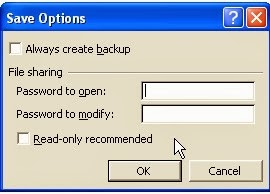13.    Printing You can easily print excel files for hard copy. You can print worksheets in the form of separate printed pages. Normally MS Excel is used to prepare proformas of various kinds. These proformas are filled with required data and then print command is applied to get the proformas printed as hard copies.14.    Recalculation

Recalculation feature means the calculations in worksheet will be automatically recalculated if you change data in worksheet. For example, let we type 10 in cell A1, 20 in cell A2 and 30 in cell A3. Now we apply Sum function in cell A4 as "=Sum(A1:A3)" and press Enter key. The answer = 10+20+30 = 60 will be displayed in A4 cell. Now if we change data in cell A1 to zero. Then the total in cell A4 will be changed to 50 instantly. Because 0+20+30 will be recalculated automatically and answer 50 will be displayed in cell A4.15.    Email worksheets

In Excel you can send worksheets by Email to one or more persons. We can send prepared worksheets in MS Excel to send by Email when computer is connected to internet.

16. Pivot Tables

A pivot table may be defined as an interactive table that is used to quickly combine and compare large amounts of data. Pivot table is an excellent feature of MS Excel especially for business organizations to summarize important data quickly and easily.